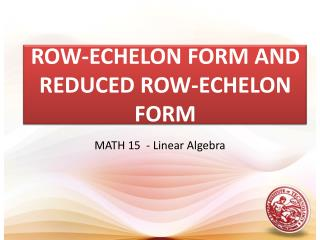DownloadDownload PresentationROW-ECHELON FORM AND REDUCED ROW-ECHELON FORM

# ROW-ECHELON FORM AND REDUCED ROW-ECHELON FORM

Télécharger la présentation## ROW-ECHELON FORM AND REDUCED ROW-ECHELON FORM

- - - - - - - - - - - - - - - - - - - - - - - - - - - E N D - - - - - - - - - - - - - - - - - - - - - - - - - - -
##### Presentation Transcript

1. ROW-ECHELON FORM AND REDUCED ROW-ECHELON FORM MATH 15 - Linear Algebra

2. DEFINITION A matrix is in Reduced Row Echelon Form (RREF) if it satisfies the following: A zero row (row containing entirely of zeroes), if it exists, appear at the bottom of the matrix The first non zero element in a row is a 1. This is known as the leading one. The next leading one appears below and to the right of the preceding leading one. The leading one is the only non zero element in its column. If A, B and C are satisfied, the matrix is in Row Echelon Form.

3. EXAMPLE Row-Echelon Form Reduced Row-Echelon Form

4. REDUCED ROW-ECHELON FORM Leading 1’s have 0’s above and below them.

5. ROW-ECHELON FORM Leading 1’s shift to the right in successive rows.

6. NOT IN ROW-ECHELON FORM Leading 1’s do not shift to the right in successive rows.

7. ROW EQUIVALENCE Two matrices are row equivalent if one can be changed to the other by a sequence of elementary row operations. Alternatively, two matrices are row equivalent if and only if they have the same row space. If a matrix can be obtained from a matrix by using a series of elementary row operations, then we say the matrices are row equivalent. This is indicated using the notation

8. ROW EQUIVALENCE An matrix is row equivalent to if it can be obtained from by a finite sequence of elementary row operations. Theorems: 1. Every matrix is equivalent to a matrix in row echelon form. 2. Every matrix is equivalent to a unique matrix in reduced row echelon form.

9. ELEMENTARY ROW OPERATIONS An elementary row operation is any one of the following moves: Swap: Swap two rows of a matrix. Scale: Multiply a row of a matrix by a nonzero constant Pivot: Add to one row of a matrix some multiple of another row.

10. ELEMENTARY ROW OPERATIONS We use the following notation to describe the elementary row operations. : Change the throw by adding times row to it, then put the result back in row . : Multiply the throw by k. : Interchange the thand throws.

11. REDUCTION TO ROW-ECHELON FORM Here is a systematic way to put a matrix in row-echelon form using elementary row operations. Start by obtaining 1 in the top left corner. Then obtain zeros below that 1 by adding appropriate multiples of the first row to the rows below it. Next, obtain a leading 1 in the next row, and then obtain zeros below that 1. At each stage make sure that every leading entry is to the right of the leading entry in the row above it−rearrange the rows if necessary. Continue this process until you arrive at a matrix in row-echelon form.

12. REDUCTION TO ROW-ECHELON FORM This is how the process might work for a 3 × 4 matrix:

13. THE SOLUTIONS OF A LINEAR SYSTEM IN ROW-ECHELON FORM No solution. If the row-echelon form contains a row that represents the equation where is not zero, then the system has no solution. A system with no solution is called inconsistent. One solution. If each variable in the row-echelon form is a leading variable, then the system has exactly one solution, which we find using back-substitution or Gauss-Jordan elimination.

14. THE SOLUTIONS OF A LINEAR SYSTEM IN ROW-ECHELON FORM Infinitely many solutions. If the variables in the row-echelon form are not all leading variables, and if the system is not inconsistent, then it has infinitely many solutions. In this case, the system is called dependent. We solve the system by putting the matrix in reduced row-echelon form and then expressing the leading variables in terms of the nonleading variables. The nonleading variables may take on any real numbers as their values.

15. THE SOLUTIONS OF A LINEAR SYSTEM IN ROW-ECHELON FORM No solution Last equation says 0 = 1. One solution Each variable is a leading variable.

16. THE SOLUTIONS OF A LINEAR SYSTEM IN ROW-ECHELON FORM Infinitely many solutions is not a leading variable.